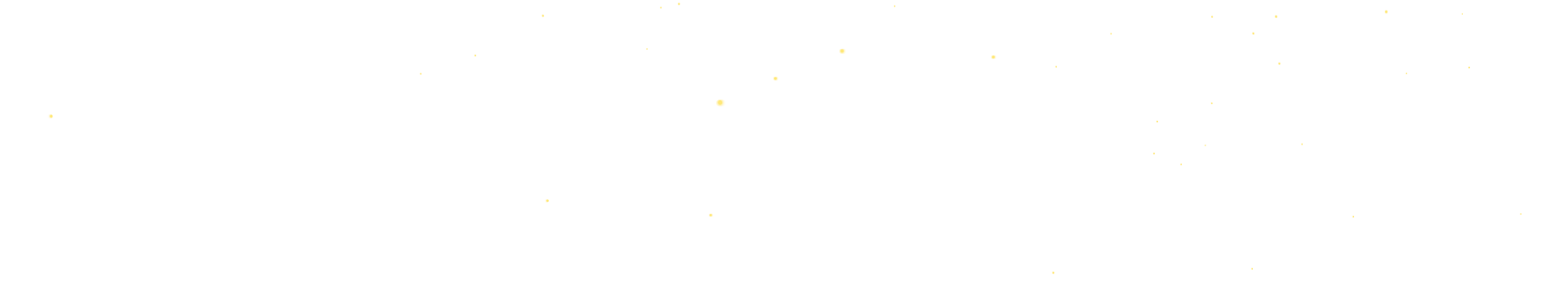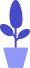# Python turtle module example: draw Peppa Pig (on)

Estimated reading: 5 minutes 64 views

In the previous tutorial, we have analyzed Peppa Pig, now let’s draw it.

## Set up canvas and brushes

First, we define a setting() function, which we use to set the canvas and brush. The code for the setting() function is shown below.

``````def setting():
setup(800,500)
pensize(4)
hideturtle()
colormode(255)
speed(10)``````

The setting() function mainly does some settings and preparations before drawing. It first sets the canvas to be 800 pixels wide and 500 pixels high. Then set the brush size to 4 and hide the little turtle icon. Call colormode(255) to set the RGB color value range from 0 to 255. Call speed(10) to set the brush speed to 10.

## nose() function

Next, we first define the function to draw the nose, the code of the function is as follows.

``````def nose():
penup()
goto(-100,100)
color((255,155,192),"pink") #The brush color is light pink, and the fill color is pink
pendown()
begin_fill()
#Draw an ellipse as the outline of the nose
segment=0.4
for i in range(120):
if 0<=i<30 or 60<=i<90:
segment= segment+0.08 #acceleration
left(3) #Turn left 3 degrees
forward(segment) #Draw a straight line
else:
segment= segment-0.08 #deceleration
left(3) #Turn left 3 degrees
forward(segment) #Draw a straight line
end_fill()
#left nostril
penup()
forward(25)
forward(10)
color((255,155,192),(160,82,45)) #The brush color is light pink, and the fill color is loess ochre
pendown()
begin_fill()
circle(5)
end_fill()
#right nostril
penup()
forward(20)
pendown()
begin_fill()
circle(5)
end_fill()``````

First call the penup() function to pick up the brush to avoid leaving traces on the canvas. Then call the goto() function to position the pen to the specified coordinates. Call setheading() to set the direction of movement of the brush when it starts. Then call the color() function, set the color of the brush to light pink, and set the color of the fill to pink, which is Page’s iconic color.

The pendown() function is then called to drop the brush, and now any movement of the brush leaves a trail. Next, we draw an ellipse. The drawing method has been introduced before and will not be described in detail here. This completes the outline of the nose.

Now let’s draw Peppa’s nostrils. Or pick up the brush, position the brush to the specified coordinates, set the direction of movement at startup, specify the brush color and fill color, and drop the brush. Then draw a circle. In this way, the left nostril is drawn, and the code for the right nostril is similar, so I won’t repeat it here. Call this function to see the drawing effect, as shown in Figure 1.figure 1

Next we define the head() function, which is used to draw the head. The code for the head() function is shown below.

``````def head():
penup()
goto(-69,167)
pendown()
color((255,155,192),"pink")
begin_fill()
circle(300,-30)
circle(100,-60)
circle(80,-100)
circle(150,-20)
circle(60,-95)
circle(-300,15)
#Outline the outline of the right half of the nose to avoid covering it when filling
penup()
goto(-100,100)
pendown()
segment=0.4
for i in range(60):
if 0<=i<30 or 60<=i<90:
segment= segment+0.08
left(3)
forward(segment)
else:
segment= segment-0.08
left(3)
forward(segment)
end_fill()``````

Pick up the brush, position the brush to the specified coordinates, and drop the brush. Specify the brush color and fill color. Call the begin_fill() function to start filling. Set the direction of motion at startup, then draw the head by drawing a few arcs.

Next, pick up the brush again and position the brush at (−100,100), which is where the nose was drawn. Then draw the outline of the right half of the nose, which is half an ellipse. This is done to avoid covering the nose when filling the head. Finally, the end_fill() function is called to end the filling.

Call this function to see its drawing effect, as shown in Figure 2.figure 2

## ears() function

Next we define the ears() function, which is used to draw the ears. The code for the ears() function is shown below.

``````def ears():
color((255,155,192),"pink")
#left ear
penup()
goto(0,160)
pendown()
begin_fill()
circle(-50,50)
circle(-10,120)
circle(-50,54)
end_fill()
#right ear
penup()
forward(-12)
forward(30)
pendown()
begin_fill()
circle(-50,50)
circle(-10,120)
circle(-50,56)
end_fill()``````

The code is similar to the previous one and will not be repeated here. Call the ears() function to see the drawing effect, as shown in Figure 3.image 3

## eyes() function

Next we define the eyes() function, which is used to draw the eyes. The code for the eyes() function is shown below.

``````def eyes():
#left eye socket
color((255,155,192),"white")
penup()
forward(-20)
forward(-95)
pendown()
begin_fill()
circle(15)
end_fill()
#left eyeball
color("black")
penup()
forward(12)
forward(-3)
pendown()
begin_fill()
circle(3)
end_fill()
#right eye frame
color((255,155,192),"white")
penup()
forward(-25)
forward(40)
pendown()
begin_fill()
circle(15)
end_fill()
#right eyeball
color("black")
penup()